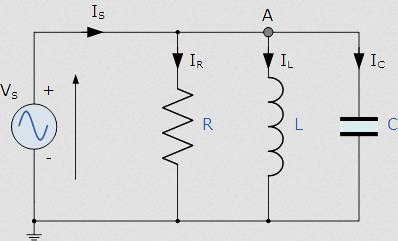Search by category:

# How to Find Calculate Resonant Frequency of Parallel RLC Circuit Formula

How to Find Calculate Resonant Frequency of Parallel RLC Circuit Formula a complete package after which its not need anything to understand it. Resonant is the property of the electronic circuit and having ability to resonate ate the specific frequency and it represents f0 and the unit of frequency is hertz. Resonant basically the process of energy storage which occurs between the two components one is known as inductor and second one is known as capacitor. Inductor stores the magnetic energy when current flow through the inductor and on the other hand the capacitor charged though electric charge when supply passes through the capacitor. In parallel RLC circuits the arrangement of the components of resistance, inductor and the capacitor always parallel to each other and then to calculate the resonance frequency effect through this circuit. Basically resonance frequency is defined as the frequency that’s impedance of the circuit is minimum and on the other hand in the resonance frequency the capacitive reactance and inductive reactance of any circuit should be equal to each other either it’s a RLC parallel or any other circuitry.Resonance occurs when XL = XC :

XL = XC

fL = 1/ 2πfC

f2 =1/ (2πL× 2πC)

f2 =1/ (4π2LC)

f =√1/ (4π2LC)

f0 = 1/ 2π √LC   (Hz)

Actually in Parallel RLC circuit the capacitor and inductor cancellation the each other effect and due to respect the only resistance will be maximum of the circuit are equal to the impedance of the circuit and that the effect will be easy to calculate the resonant frequency of the circuit.